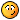### Accretion Energy

I compute here the gravitational energy released in the assembly of material from infinity to form a spherical ruble pile. This is also the energy required to disperse it again.

Let ρ be the pile’s constant density. Let m be the mass of the pile which will increase as the pile accretes. When a new mass element, dm, falls in from infinity the attraction is f = G m dm/r2 while the element is still at distance r. The volume of the pile is (4/3)πr03 = m/ρ where r0 is the pile’s radius.
It will reach the surface at radius r0 = ((4/3)πρ/m)−1/3 having released energy = de =
r0f dr = ∫r0G m dm r−2 dr = G m dm/r0 = ((4/3)πρ/m)1/3 G m dm = G dm ((4/3)πρ)1/3 m2/3.
As the mass increases the total energy is
0Mde = ∫0MG dm ((4/3)πρ)1/3 m2/3 = G ((4/3)πρ)1/30M m2/3 dm
= G ((4/3)πρ)1/3 (3/5)M5/3 = 22/332/35−1 (πρ)1/3G M5/3 = 62/3/5 (πρ)1/3G M5/3
where M is the total mass of the pile.
This agrees almost with this.

Expressing the answer in M and the radius r using M = (4π/3)r3ρ
ρ = M(3/(4π))r−3
E = 62/3/5 (πρ)1/3G M5/3
= 62/3/5 (πM(3/(4π))r−3)1/3G M5/3
= 22/3−2/332/3+1/3/5π0M6/3G r−1
= 3/5M2G/r
= 0.6 M2G/r
= (4.0044 10−11 M2/r) m3 kg−1 s−2

Holding the density constant, the energy varies as the 5th power of the radius.

Accretion Energy of Earth:
Mass = 5.98 × 1024 kg.
Radius of Earth = 6.37 × 106 m.
G = 6.6734∙10−10 m3kg−1sec−2
E = 2.248 × 1032 Joules

P.S., Today, 2007 July 24, I fixed a bug that crept in as I expressed E in terms of M and r. These calculations are trivial yet very error prone for me. My old equation had the wrong units.I need a calculator that does units!! (So does NASA!)
(If you plan to disassemble the Earth you should first check my calculations.)

And on 2008 Nov 10 I found and fixed another bug. Very embarrassing!

A neutron star of radius 5 km and density 1015 g cm−3 would have a mass of (4/3) π (5 km)3 1015 g cm−3 (105 cm/km)3
= (500/3) π 1030 g
= 5.24 1032 g.

By the above formula the accretion energy is (4.004 10−11 27.5 1064 g2 / (5 103 m)) m3 kg−1 s−2 (103 g/kg)−2
= 22 1051 kg (m/s)2 = 2.2 1052 Joules.
This does not include the substantial energy that is required to overcome degeneracy pressure.